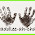Pages

Mandala - Six Segement vs Eight Segment

After recreating several mandalas it becomes obvious that there are two basic techniques for the beginning steps. Circles can be easily divided into four segments and six segments. Therefore, mandalas are frequently radially symmetric with three, six or twelve matching points of rotation or four, eight or sixteen matching points of rotation. Knowing if the mandala is based on a multiple of four or six determines the starting technique.

Mandalas which contain four or eight segments typically begin following the steps shown in the creation of this mandala. Basically a circle is drawn with one diameter. A perpendicular bisector is drawn through the diameter creating four 90 degree angles. The angles are then bisected again to create eight 45 degree angles.

A second technique, discussed below, is used when circles are divided into multiples of six segments.

This mandala contains twelve small circles surrounding the center circle.

To divide a circle into six segments first draw the main circle, once the circle is drawn, keep the radius set to the circle radius, place the compass point anywhere on the circumference of the circle and create tick marks where the compass crosses the main circle. Then move the compass point to each tick mark and create another tick mark where the compasses crosses the circle. Continue until there are six tick marks. This divides the circle into six equal parts. The six angles can be further bisected into twelve parts.

Once the circle is divided into twelve parts, the twelve angles can be bisected. Instead of drawing lines, tick marks were placed where the bisectors crossed the circle in the above photo. With the tick marks locating the center points for each of the twelve circles.

Finally, the compass was widened to match the distance from the center to the tangent points on the radial twelve circles.

Counting the points of symmetry and determining whether there is a multiple of six or four determines the starting point for many mandalas created with a straight edge and compass.

Other Mandala construction tutorials are available on our Math Page.Check out these great blogs full of educational activity ideas.

1 comment:

1.Thank you for sharing! This is definitely a math project we would like to incorporate into our fun homeschool Fridays!